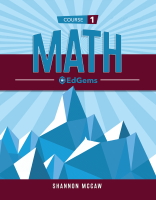1 Unit 1 Ratios and Rates 1.1:  Ratios Also consider: 1.1:  Ratios Also consider: 1.2:  Ratio Tables and Graphs Also consider: 1.2:  Ratio Tables and Graphs Also consider: 1.3:  Rates and Unit Rates 1.3:  Rates and Unit Rates 1.4:  Comparing Rates 1.4:  Comparing Rates 1.5:  Measurement Conversions Also consider: 1.5:  Measurement Conversions Also consider: 1.6:  Circumference and Pi 1.6:  Circumference and Pi
 2 Unit 2 Multi-Digit Operations 2.1:  Adding and Subtracting Decimals Also consider: 2.1:  Adding and Subtracting Decimals Also consider: 2.2:  Multiplying Decimals Also consider: 2.2:  Multiplying Decimals Also consider: 2.3:  Dividing by 1-Digit Numbers 2.3:  Dividing by 1-Digit Numbers 2.4:  Dividing by Multi-Digit Numbers 2.4:  Dividing by Multi-Digit Numbers 2.5:  Dividing Decimals 2.5:  Dividing Decimals 2.6:  Common Factors and Multiples 2.6:  Common Factors and Multiples
 3 Unit 3 Percents 3.1:  Introducing Percents 3.1:  Introducing Percents 3.2:  Percents, Decimals, and Fractions Also consider: 3.2:  Percents, Decimals, and Fractions Also consider: 3.3:  Percent of a Number 3.3:  Percent of a Number 3.4:  Percent Applications Also consider: 3.4:  Percent Applications Also consider:
 4 Unit 4 Fraction Operations 4.1:  Multiplying Fractions Also consider: 4.1:  Multiplying Fractions Also consider: 4.2:  Dividing Fractions with Models •Coming soon: Divide two fractions using models 4.2:  Dividing Fractions with Models •Coming soon: Divide two fractions using models 4.3:  Dividing Fractions 4.3:  Dividing Fractions 4.4:  Multiplying and Dividing Mixed Numbers 4.4:  Multiplying and Dividing Mixed Numbers
 5 Unit 5 Expressions 5.1:  Powers and Exponents Also consider: 5.1:  Powers and Exponents Also consider: 5.2:  Order of Operations Also consider: 5.2:  Order of Operations Also consider: 5.3:  Variables and Expressions Also consider: 5.3:  Variables and Expressions Also consider: 5.4:  Evaluating Expressions Also consider: 5.4:  Evaluating Expressions Also consider: 5.5:  Equivalent Expressions Also consider: 5.5:  Equivalent Expressions Also consider: 5.6:  The Distributive Property •Coming soon: Identify equivalent numerical expressions 5.6:  The Distributive Property •Coming soon: Identify equivalent numerical expressions
 6 Unit 6 One-Variable Equations 6.1:  Equations and Solutions 6.1:  Equations and Solutions 6.2:  Solving Addition and Subtraction Equations 6.2:  Solving Addition and Subtraction Equations 6.3:  Solving Multiplication and Division Equations 6.3:  Solving Multiplication and Division Equations 6.4:  Solving Percent Equations Also consider: 6.4:  Solving Percent Equations Also consider:
 7 Unit 7 Rational Numbers and the Coordinate Plane 7.1:  Understanding Integers Also consider: 7.1:  Understanding Integers Also consider: 7.2:  Comparing Rational Numbers Also consider: 7.2:  Comparing Rational Numbers Also consider: 7.3:  Inequalities •Coming soon: Write and graph inequalities: word problems Also consider: 7.3:  Inequalities •Coming soon: Write and graph inequalities: word problems Also consider: 7.4:  The Coordinate Plane Also consider: 7.4:  The Coordinate Plane Also consider: 7.5:  Quadrilaterals and the Coordinate Plane Also consider: 7.5:  Quadrilaterals and the Coordinate Plane Also consider:
 8 Unit 8 Two-Variable Equations 8.1:  Input-Output Tables 8.1:  Input-Output Tables 8.2:  Writing Two-Variable Equations 8.2:  Writing Two-Variable Equations 8.3:  Graphing Two-Variable Equations 8.3:  Graphing Two-Variable Equations
 9 Unit 9 Area and Volume 9.1:  Area with Fractions 9.1:  Area with Fractions 9.2:  Area and Perimeter with Decimals 9.2:  Area and Perimeter with Decimals 9.3:  Area of Composite Figures •Coming soon: Area of compound figures: word problems Also consider: 9.3:  Area of Composite Figures •Coming soon: Area of compound figures: word problems Also consider: 9.4:  Nets and Surface Area 9.4:  Nets and Surface Area 9.5:  Volume of Rectangular Prisms •Coming soon: Volume of compound figures 9.5:  Volume of Rectangular Prisms •Coming soon: Volume of compound figures
 10 Unit 10 Statistics 10.1:  Introduction to Statistics 10.1:  Introduction to Statistics 10.2:  Measures of Center Also consider: 10.2:  Measures of Center Also consider: 10.3:  Dot Plots Also consider: 10.3:  Dot Plots Also consider: 10.4:  Histograms Also consider: 10.4:  Histograms Also consider: 10.5:  Box-and-Whisker Plots 10.5:  Box-and-Whisker Plots 10.6:  Analyzing Statistics •Coming soon: Choose appropriate measures to describe distributions 10.6:  Analyzing Statistics •Coming soon: Choose appropriate measures to describe distributions 10.7:  Mean Absolute Deviation 10.7:  Mean Absolute Deviation
This document includes the IXL® skill alignments to EdGems MS Math's EdGems Math curriculum. IXL provides skill alignments as a service to teachers, students, and parents. The skill alignments are provided by IXL and are not affiliated with, sponsored by, reviewed, approved or endorsed by EdGems MS Math or any other third party. IXL® and IXL Learning® are registered trademarks of IXL Learning, Inc. All other intellectual property rights (e.g., unregistered and registered trademarks and copyrights) are the property of their respective owners.